# Basic Tutorial Lesson 10: Building a Shift Register Using D Flip-Flops

 Tutorial Project: Exploring Flip-Flops and Sequential Logic CircuitsObjective: In this project, you will learn about D flip-flops and will use them to build a shift register digital circuit. Concepts/Features: D Flip-Flop Propagation Delay Digital Timing Diagram Shift Register Minimum Version Required: All versions

## What You Will Learn

In this tutorial lesson you will examine the D flip-flop device and will use four D flip-flops to design a 4-bit shift register. You will use the live timing diagrams to analyze the performance of your digital circuit.

## Testing a D Flip-Flop

The following is a list of parts needed for this part of the tutorial lesson:

Part Name Part Type Part Value
In1 Digital Input 1-bit
Out1 Digital Output N/A
CLK Digital Clock Period = 100ns, Pulse Width = 50ns
A1 D-Type Flip-Flop Defaults

You will build a synchronous circuit with a digital clock and a single input as shown in the figure below. The keyboard shortcut for digital clock is Alt+C. Set the "Period" of the clock to 100ns and its "Pulse Width" to 50ns. Connect the digital input device to the "D" pin of the flip-flop and the digital output device to its "Q" pin. The D flip-flop transfers the input data at its input to its Q output on the rising edge of the clock pulse. During the rest of the clock cycle, the output remains unchanged (hold state).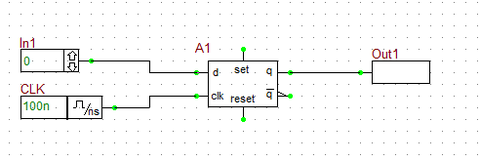The D-Type Flip-Flop device of RF.Spice A/D.

To understand the operation of the flip-flop circuit, you will use the "Live Digital Timing Diagram" feature of RF.Spice A/D. As you earned in the previous tutorial lesson, set the step time to 20ns. Set the input initially to In1 = 0. Then, to activate the timing diagram, click the "Show/Hide Live Digital Timing Diagrams"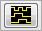button of the Schematic Toolbar. Remember that nothing will happen until you start a digital simulation. Click the "Step"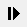button of the Main Toolbar or use the keyboard shortcut Ctrl+H to advance the simulation time one step at a time. Step the simulation time to 60ns and then change the input to In1 = 1. Keep stepping to 160ns and then change the input to In1 = 0 again. Continue stepping to 400ns and change the input to In1 = 1 once again and then proceed to 600ns. The table below summarizes the input entries:

Time In1
0ns 0
160ns 1
260ns 0
400ns 1

The timing diagram of your D flip-flop circuit is shown in the figure below. As you can see from the figure, the input first rises from 0 to 1 at t = 160ns. The flip-flop waits until the next rising edge of the clock signal at t = 200ns. The input's high state is transferred to the output Q with a propagation delay of 14ns. According to the property dialog of the D Flip-Flop device, the low-to-high propagation delay of the device is tPLH = 14ns, while its high-to-low propagation delay is tPHL = 1420ns. Therefore, Q rises from 0 to 1 at t = 214ns and stays high for the next several clock cycles. The input next falls from 1 to 0 at t = 260ns. The flip-flop waits until the next rising edge of the clock signal at t = 300ns. The input's low state is transferred to the output Q with a propagation delay of 20ns. Therefore, Q falls from 1 to 0 at t = 320ns and stays low for the next several clock cycles. The input rises again from 0 to 1 at t = 400ns and stay high for the rest of the simulation time. This time the flip-flop has to wait a full clock period until the next rising edge of the clock signal at t = 500ns. The input's high state is transferred to the output Q with a propagation delay of 14ns. Then, Q rises again from 0 to 1 at t = 514ns and stays high for the rest of the simulation time.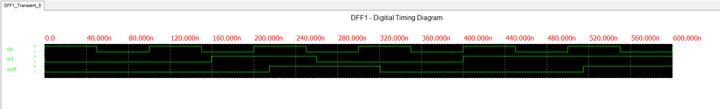The digital timing diagram of the single D-Type Flip-Flop circuit.

## Building a 4-Bit Shift Register Using D Flip-Flops

The following is a list of parts needed for this part of the tutorial lesson:

Part Name Part Type Part Value
Data Digital Input 1-bit
B0 - B3 Digital Output N/A
CLK Digital Clock Period = 100ns, Pulse Width = 50ns
A1 - A4 D-Type Flip-Flop Defaults

A shift register is a multi-output digital circuit that transfer the input data to its outputs sequentially at each clock cycle. Cascade the four D flip-flops as shown on the figure by connecting the Q pin of each to the D pin of the next. All four flip-flops share the same clock signal. Use the same clock settings from the previous part (Period = 100ns and Pulse Width = 50ns). Set the step time to 20ns. Enable the live digital timing diagram. Run a digital simulation and step through 1200ns. Set the value of the input "Data" to 0 and change it to 1 at t = 100ns and change it back to 0 at t = 600ns.

Your timing diagram will look like the figure below. As you can see from the figure, after the input is set high at t = 100ns for the first time, it takes the first flip-flop until the clock's next rising edge at t = 200ns to react. The first output B0 rises to 1 at t = 214ns (tPLH = 14ns). As the clock cycles proceed, the other outputs B1, B2 and B3, sequentially rise to 1 at t = 314ns, 414ns, and 514ns, respectively. All four outputs remain high for the next several clock cycles (at hold state).

The input "Data" next falls from 1 to 0 at t = 600ns. It takes the first flip-flop until the clock's next rising edge at t = 700ns to react. The first output B0 fall from to 0 at t = 720ns (tPHL = 20ns). As the clock cycles proceed, the other outputs B1, B2 and B3, sequentially fall to 0 at t = 820ns, 902ns, and 1020ns, respectively. All four outputs remain low afterwards (at hold state).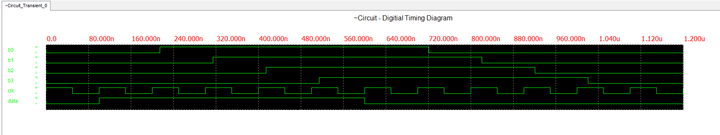The timing diagram of the 4-bit shift register circuit.